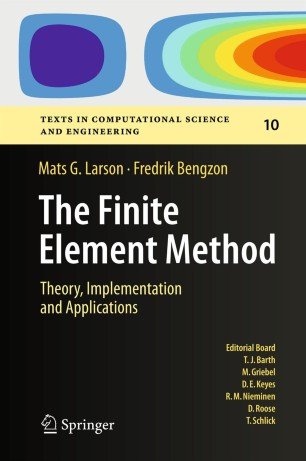# The Finite Element Method: Theory, Implementation, and ApplicationsTextbook

Part of the Texts in Computational Science and Engineering book series (TCSE, volume 10)

1. Front Matter
Pages i-xvii
2. Mats G. Larson, Fredrik Bengzon
Pages 1-22
3. Mats G. Larson, Fredrik Bengzon
Pages 23-44
4. Mats G. Larson, Fredrik Bengzon
Pages 45-69
5. Mats G. Larson, Fredrik Bengzon
Pages 71-111
6. Mats G. Larson, Fredrik Bengzon
Pages 113-142
7. Mats G. Larson, Fredrik Bengzon
Pages 143-176
8. Mats G. Larson, Fredrik Bengzon
Pages 177-201
9. Mats G. Larson, Fredrik Bengzon
Pages 203-223
10. Mats G. Larson, Fredrik Bengzon
Pages 225-239
11. Mats G. Larson, Fredrik Bengzon
Pages 241-256
12. Mats G. Larson, Fredrik Bengzon
Pages 257-287
13. Mats G. Larson, Fredrik Bengzon
Pages 289-325
14. Mats G. Larson, Fredrik Bengzon
Pages 327-354
15. Mats G. Larson, Fredrik Bengzon
Pages 355-372
16. Back Matter
Pages 373-385

### Introduction

This book gives an introduction to the finite element method as a general computational method for solving partial differential equations approximately. Our approach is mathematical in nature with a strong focus on the underlying mathematical principles, such as approximation properties of piecewise polynomial spaces, and variational formulations of partial differential equations, but with a minimum level of advanced mathematical machinery from functional analysis and partial differential equations. In principle, the material should be accessible to students with only knowledge of calculus of several variables, basic partial differential equations, and linear algebra, as the necessary concepts from more advanced analysis are introduced when needed. Throughout the text we emphasize implementation of the involved algorithms, and have therefore mixed mathematical theory with concrete computer code using the numerical software MATLAB is and its PDE-Toolbox. We have also had the ambition to cover some of the most important applications of finite elements and the basic finite element methods developed for those applications, including diffusion and transport phenomena, solid and fluid mechanics, and also electromagnetics.​

### Keywords

adaptive finite element methods applications of finite elements error estimates finite element method implementation of finite elements

#### Authors and affiliations

1. 1., Department of MathematicsUmeå UniversityUmeaSweden
2. 2., Department of MathematicsUmeå UniversityUmeaSweden

### Bibliographic information

• Book Title The Finite Element Method: Theory, Implementation, and Applications
• Authors Mats G. Larson
Fredrik Bengzon
• Series Title Texts in Computational Science and Engineering
• DOI https://doi.org/10.1007/978-3-642-33287-6
• Copyright Information Springer-Verlag Berlin Heidelberg 2013
• Publisher Name Springer, Berlin, Heidelberg
• eBook Packages Mathematics and Statistics Mathematics and Statistics (R0)
• Hardcover ISBN 978-3-642-33286-9
• Softcover ISBN 978-3-642-44711-2
• eBook ISBN 978-3-642-33287-6
• Series ISSN 1611-0994
• Edition Number 1
• Number of Pages XVII, 395
• Number of Illustrations 0 b/w illustrations, 0 illustrations in colour
• Topics
• Buy this book on publisher's site
Industry Sectors
Electronics
IT & Software
Energy, Utilities & Environment
Aerospace
Oil, Gas & Geosciences
Engineering

## Reviews

From the reviews:

“The authors give an introduction to the finite element method as a general computational method for solving partial differential equations (PDEs) approximately. … The book should be accessible to students with only knowledge of calculus of several variables, basic partial differential equations, and linear algebra, as the necessary concepts from more advanced analysis are introduced when needed.” (Răzvan Răducanu, Zentralblatt MATH, Vol. 1263, 2013)